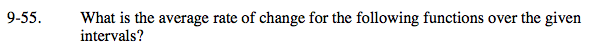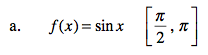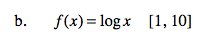### Home > PCT > Chapter Ch9 > Lesson 9.1.4 > Problem9-55

9-55.$\frac{\sin(\pi) - \sin\left(\frac{\pi}{2}\right)}{\pi - \frac{\pi}{2}} = ?$$\frac{f(10) - f(1)}{10 - 1} = ?$

log(1) = 0